# A beginner's guide to R ProgrammingIn this post, you'll learn R Programming for beginner

In this post, you'll learn R Programming for beginner

## Introduction

R is a programming language focused on statistical and graphical analysis. It is therefore commonly used in statistical inference, data analysis and Machine Learning. R is currently one of the most requested programming language in the Data Science job market (Figure 1).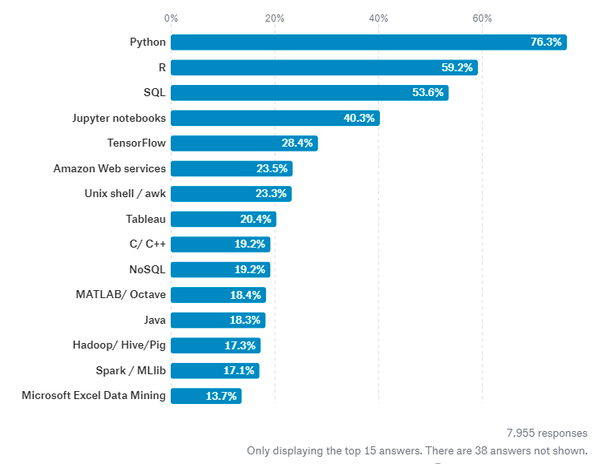Figure 1: Most Requested programming languages for Data Science in 2019 

R is available to be installed from r-project.org and one of R most commonly used integrated development environment (IDE) is certainly RStudio.

There are two main types of packages (libraries) which can be used to add functionalities to R: base packages and distributed packages. Base packages come with the installation of R, distributed packages can instead be downloaded for free using CRAN.

Once installed R, we can then get started doing some data analysis!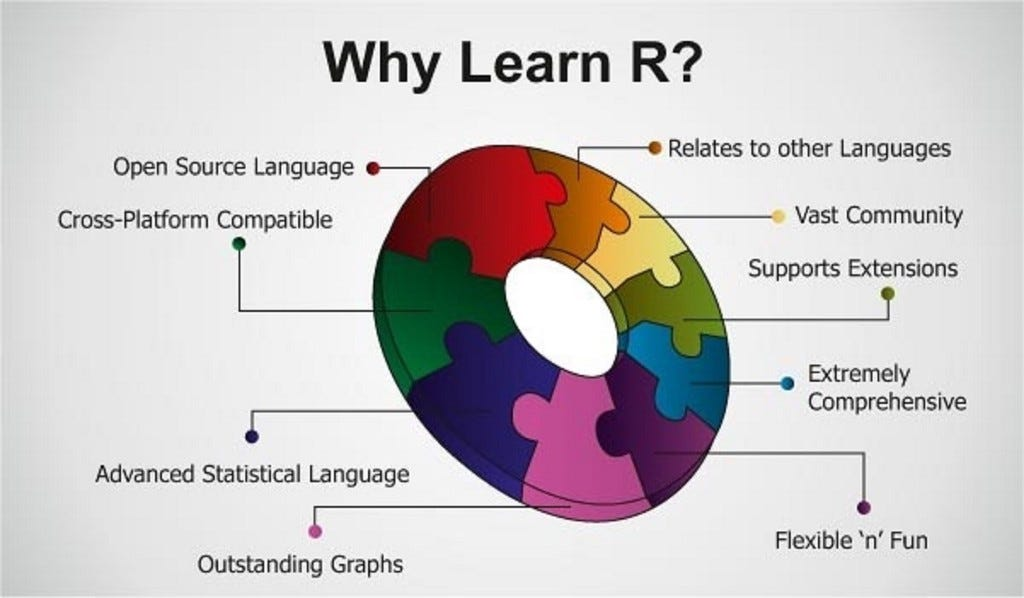## Demonstration

In this example, I will walk you through an end to end analysis of the Mobile Price Classification Dataset to predict the price range of Mobile Phones. The code I used for this demonstration is available on both my GitHub and Kaggle account.

Importing Libraries

First of all, we need to import all the necessary libraries.

Packages can be installed in R using the install.packages() command and then loaded using the library() command. In this case, I decided to install first PACMAN (Package Management Tool) and then use it to install and load all the other packages. PACMAN makes loading library easier because it can install and load all the necessary libraries in just one line of code.

``````install.packages("pacman")
library(pacman)
pacman::p_load(pacman, dplyr, ggplot2, rio, gridExtra, scales, ggcorrplot, caret, e1071)
``````

The imported packages are used to add the following functionalities:

• *dplyr: *data processing and analysis.
• *ggplot2: *data visualization.
• *rio: *data import and export.
• *gridExtra: *to make plots graphical objects to which can be freely arranged on a page.
• *scales: *used to scale data in plots.
• ggcorrplot: **to **visualize correlation matrices using ggplot2 in the backend.
• caret: **is used to **train and plot classification and regression models.
• *e1071: *contains functions to perform Machine Learning algorithms such as Support Vector Machines, Naive Bayes, etc…

Data Pre-processing

We can now go on loading our dataset, displaying it’s first 5 columns (Figure 2) and print a summary of the main characteristics of each feature (Figure 3). In R, we can create new objects using the *<-* operator.

``````# Loading our dataset
df <- import("./mobile_price.csv")

# Getting set of descriptive statistics, depending on the type of variable.
# In case of a Numerical Variable -> Gives Mean, Median, Mode, Range and Quartiles.
# In case of a Factor Variable -> Gives a table with the frequencies.
# In case of Factor + Numerical Variables -> Gives the number of missing values.
# In case of character variables -> Gives the length and the class.
summary(df)
``````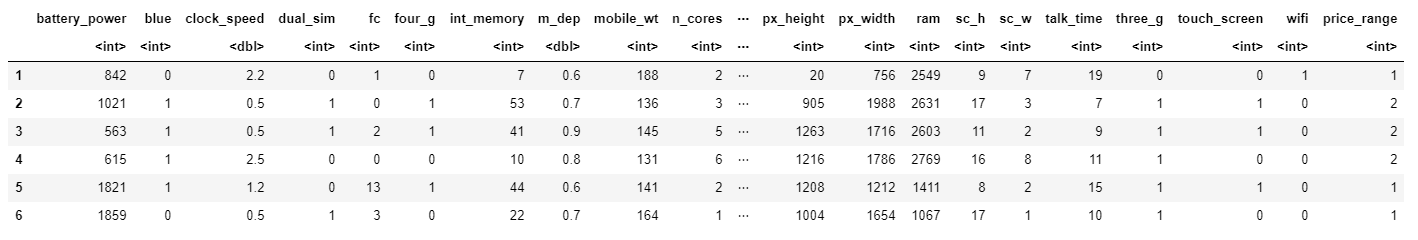The summary function provides us with a brief statistical description of each feature in our dataset. Depending on the nature of the feature in consideration, different statistics will be provided:

• *dplyr: *data processing and analysis.
• *ggplot2: *data visualization.
• *rio: *data import and export.
• *gridExtra: *to make plots graphical objects to which can be freely arranged on a page.
• *scales: *used to scale data in plots.
• ggcorrplot: **to **visualize correlation matrices using ggplot2 in the backend.
• caret: **is used to **train and plot classification and regression models.
• *e1071: *contains functions to perform Machine Learning algorithms such as Support Vector Machines, Naive Bayes, etc…

Factors are a type of data object used in R to categorize and store data (eg. integers or strings) as levels. They can, for example, be used to one hot encode a feature or to create Bar Charts (as we will see later on). Therefore they are especially useful when working with columns with few unique values.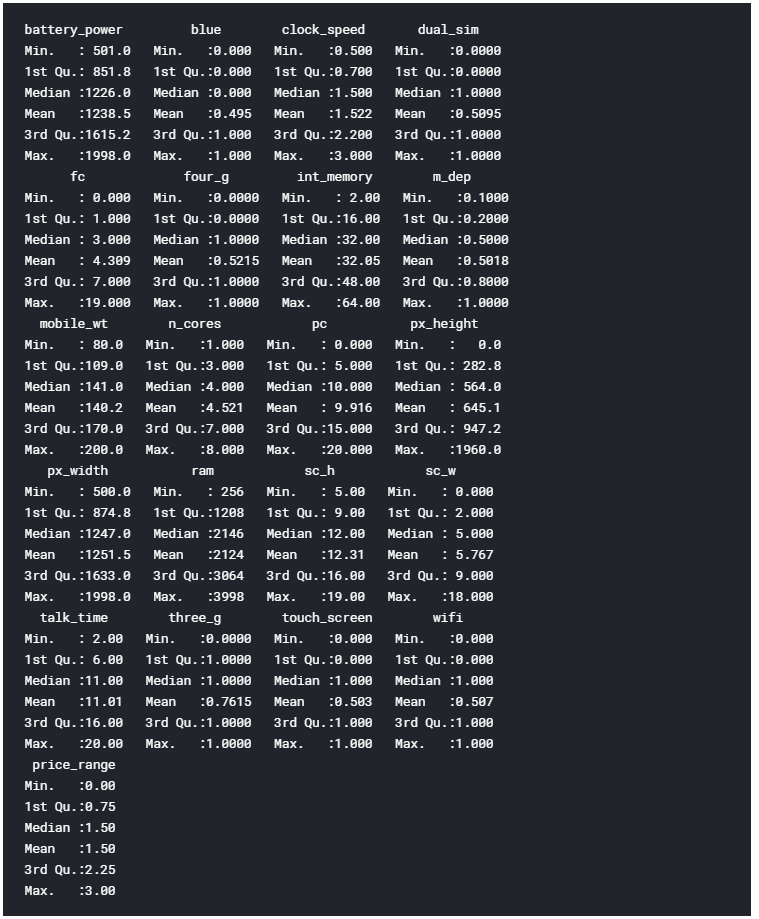Figure 3: Dataset Summary

Finally, we can now check if our Dataset contains any Not A Numbers (NaNs) value using the code shown below.

``````# Checking for Missing values
missing_values <- df %>% summarize_all(funs(sum(is.na(.))/n()))
missing_values <- gather(missing_values, key="feature", value="missing_pct")
missing_values %>%

ggplot(aes(x=reorder(feature,-missing_pct),y=missing_pct)) +

geom_bar(stat="identity",fill="red")+

coord_flip()+theme_bw()
``````

As we can see from Figure 4, no missing numbers have been found.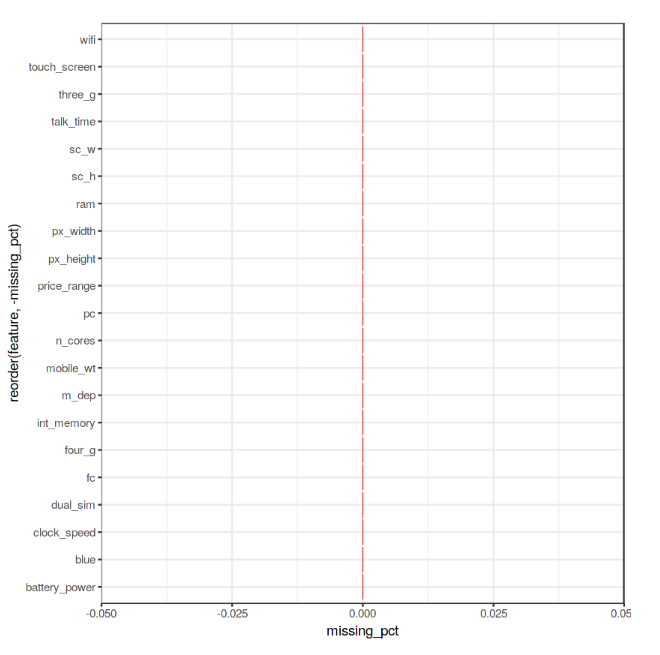Figure 4: Percentage of NaNs in each feature

Data Visualization

We can now start our Data Visualization by plotting a Correlation Matrix of our dataset (Figure 5).

``````corr <- round(cor(df), 8)
ggcorrplot(corr)
``````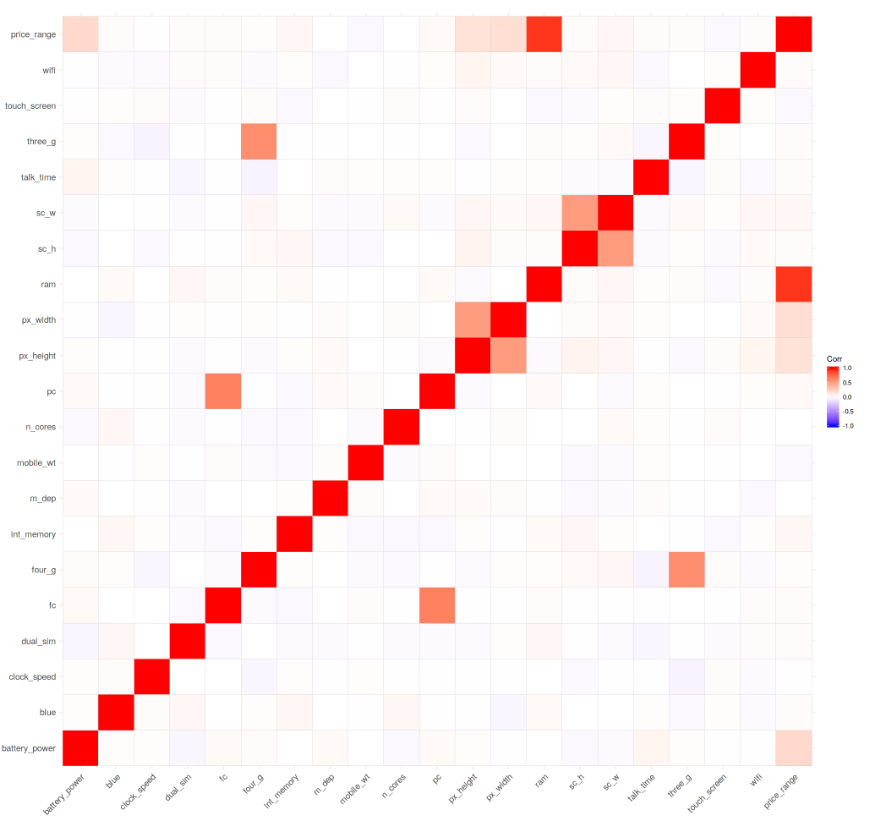Figure 5: Correlation Matrix

Successively, we can start analysing individual features using Bar and Box plots. Before creating these plots, we need though to first convert the considered features from Numeric to Factor (this allow us to bin our data and then plot the binned data).

``````df\$blue <- as.factor(df\$blue)
df\$dual_sim <- as.factor(df\$dual_sim)
df\$four_g <- as.factor(df\$four_g)
df\$price_range <- as.factor(df\$price_range)
``````

We can now create 3 Bar Plots by storing them in there different variables (p1, p2, p3) and then add them to grid.arrange() to create a subplot. In this case, I decided to examine the Bluetooth, Dual Sim and 4G features. As we can see from Figure 6, a slight majority of mobiles considered in this Dataset does not support Bluetooth, is Dual Sim and has 4G support.

``````# Bar Chart Subplots
p1 <-  ggplot(df, aes(x=blue, fill=blue)) +
theme_bw() +
geom_bar() +
ylim(0, 1050) +
labs(title = "Bluetooth") +
scale_x_discrete(labels = c('Not Supported','Supported'))
p2 <- ggplot(df, aes(x=dual_sim, fill=dual_sim)) +
theme_bw() +
geom_bar() +
ylim(0, 1050) +
labs(title = "Dual Sim") +
scale_x_discrete(labels = c('Not Supported','Supported'))
p3 <- ggplot(df, aes(x=four_g, fill=four_g)) +
theme_bw() +
geom_bar() +
ylim(0, 1050) +
labs(title = "4 G") +
scale_x_discrete(labels = c('Not Supported','Supported'))
grid.arrange(p1, p2, p3, nrow = 1)
``````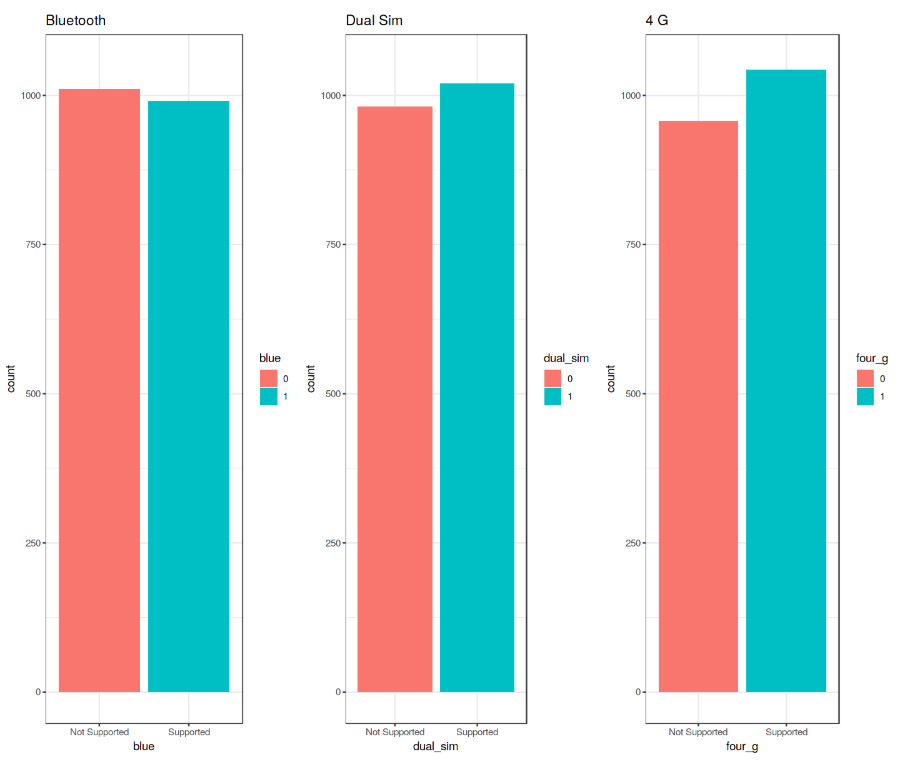Figure 6: Bar Plot Analysis

These plots have been created using R ggplot2 library. When calling the ggplot() function, we create a coordinate system on which we can add layers on top of it .

The first argument we give to the ggplot() function is the dataset we are going to use and the second one is instead an aesthetic function in which we define the variables we want to plot. We can then go on adding other additional arguments such us defining a desired geometric function (eg. barplot, scatter, boxplot, histogram, etc…), adding a plot theme, axis limits, labels, etc…

Taking our analysis a step further, we can now calculate the precise percentages of the difference between the different cases using the prop.table() function. As we can see from the resulting output (Figure 7), 50.5% of the considered mobile devices do not support Bluetooth, 50.9% is Dual Sim and 52.1% has 4G.

``````prop.table(table(df\$blue)) # cell percentages
prop.table(table(df\$dual_sim)) # cell percentages
prop.table(table(df\$four_g)) # cell percentages
``````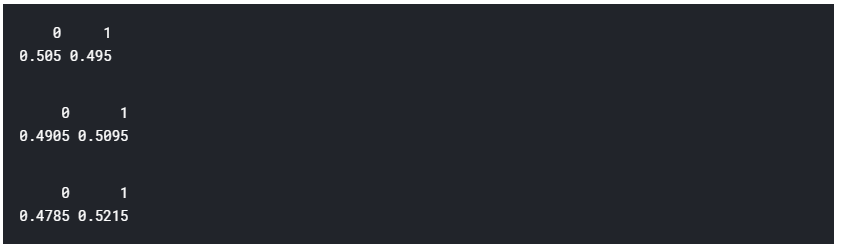Figure 7: Classes Distribution Percentage

We can now go on creating 3 different Box Plots using the same technique used before. In this case, I decided to examine how having more battery power, phone weight and RAM (Random Access Memory) can affect mobiles prices. In this Dataset, we are not given the actual phone prices but a price range indicating how high the price is (four different levels from 0 to 3).

``````# Bar Chart Subplots
p1 <-  ggplot(df, aes(x=price_range, y = battery_power, color=price_range)) +
geom_boxplot(outlier.colour="red", outlier.shape=8,
outlier.size=4) +
labs(title = "Battery Power vs Price Range")
p2 <- ggplot(df, aes(x=price_range, y = mobile_wt, color=price_range)) +
geom_boxplot(outlier.colour="red", outlier.shape=8,
outlier.size=4) +
labs(title = "Phone Weight vs Price Range")
p3 <- ggplot(df, aes(x=price_range, y = ram, color=price_range)) +
geom_boxplot(outlier.colour="red", outlier.shape=8,
outlier.size=4) +
labs(title = "RAM vs Price Range")
grid.arrange(p1, p2, p3, nrow = 1)
``````

The results are summarised in Figure 8. Increasing Battery Power and RAM consistently lead to an increase in Price. Instead, more expensive phones seem to be overall more lightweight. In the RAM vs Price Range plot have interestingly been registred some outliers values in the overall distribution.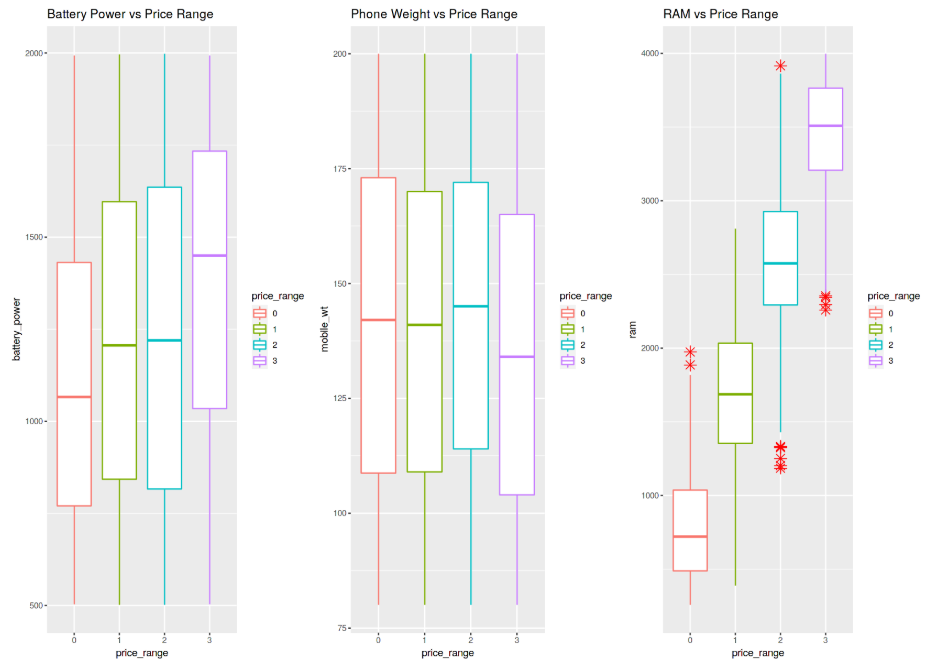Figure 8: Box Plot Analysis

Finally, we are now going to examine the distribution of camera quality in Megapixels for both the Front and Primary cameras (Figure 9). Interestingly, the Front camera distribution seems to follow an exponentially decaying distribution while the Primary camera roughly follows a uniform distribution.

``````data = data.frame(MagaPixels = c(df\$fc, df\$pc),
Camera = rep(c("Front Camera", "Primary Camera"),
c(length(df\$fc), length(df\$pc))))
ggplot(data, aes(MagaPixels, fill = Camera)) +
geom_bar(position = 'identity', alpha = .5)
``````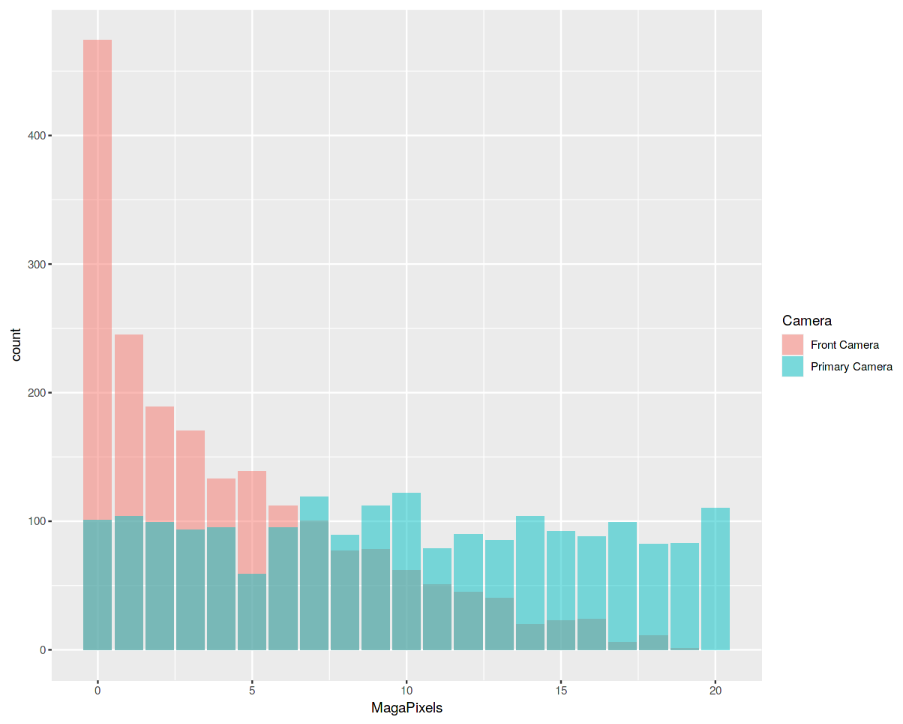Figure 9: Histogram Analysis

Machine Learning

In order to perform our Machine Learning analysis, we need first to convert our Factor variables in Numeric form and then divide our dataset into train and test sets (75:25 ratios). Lastly, we divide the train and test sets into features and labels (price_range).

``````df\$blue <- as.numeric(df\$blue)
df\$dual_sim <- as.numeric(df\$dual_sim)
df\$four_g <- as.numeric(df\$four_g)
df\$price_range <- as.numeric(df\$price_range)

## 75% of the sample size
smp_size <- floor(0.75 * nrow(df))

# set the seed to make our partition reproducible
set.seed(123)
train_ind <- sample(seq_len(nrow(df)), size = smp_size)

train <- df[train_ind, ]
test <- df[-train_ind, ]

x_train <- subset(train, select = -price_range)
y_train <- train\$price_range
x_test <- subset(test, select = -price_range)
y_test <- test\$price_range
``````

It’s now time to train our Machine Learning model. In this example, I decided to use Support Vector Machines (SVM) as our multiclass classifier. Using R summary() we can then inspect the parameters of our trained model (Figure 10).

``````model <- svm(x_train, y_train, type = 'C-classification',
kernel = 'linear')

print(model)
summary(model)
``````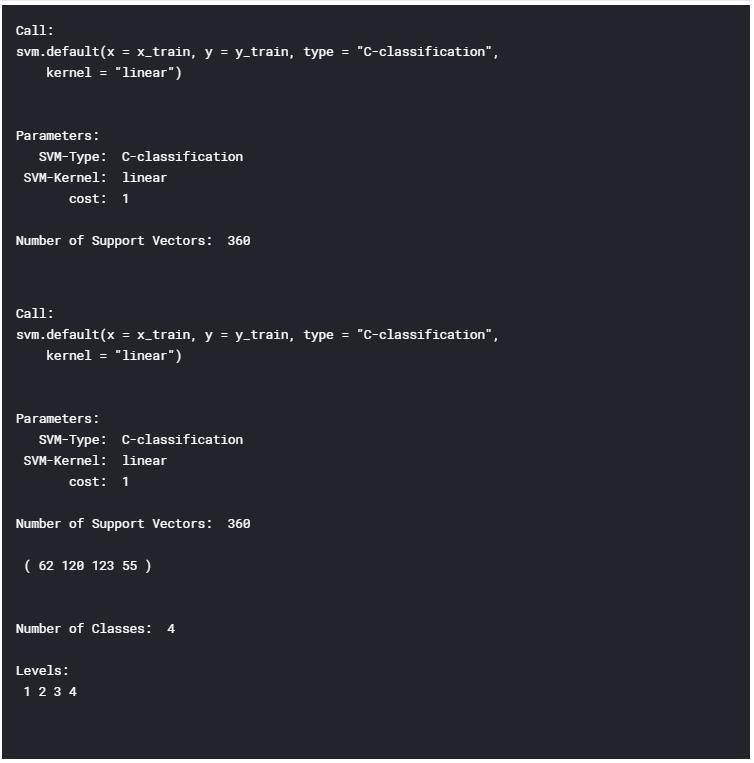Figure 10: Machine Learning Model Summary

Finally, we can now test our model making some predictions on the test set. Using R confusionMatrix() function we can then get a complete report of our model accuracy (Figure 11). In this case, an Accuracy of 96.6% was registered.

``````# testing our model
pred <- predict(model, x_test)

pred <- as.factor(pred)
y_test <- as.factor(y_test)
confusionMatrix(y_test, pred)
``````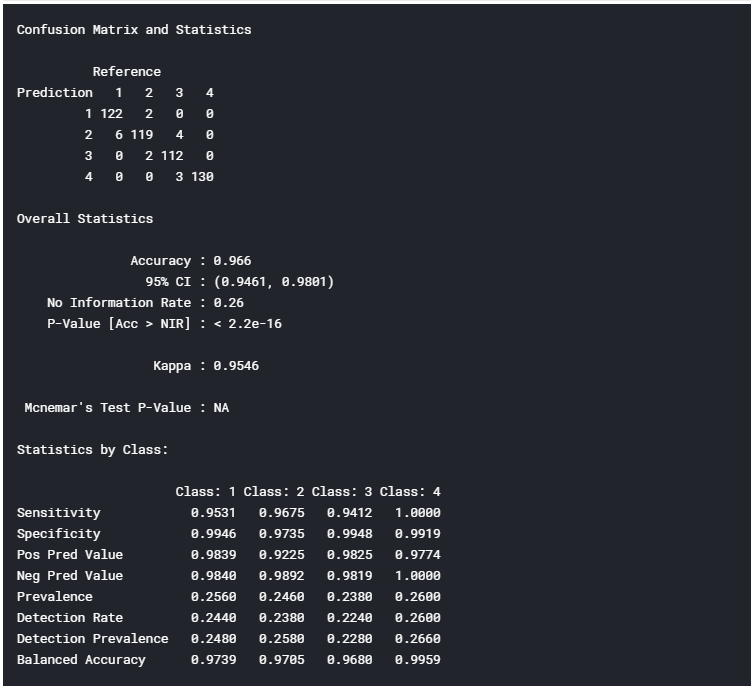Figure 11: Model Accuracy Report

If you liked this post, share it with all of your programming buddies!

## Why You Should Learn R — Learn Data Science with Dataquest

Why should you learn R programming when you're aiming to learn data science? Here are six reasons why R is the right language for you.

## Most popular Data Science and Machine Learning courses — July 2020

Most popular Data Science and Machine Learning courses — August 2020. This list was last updated in August 2020 — and will be updated regularly so as to keep it relevant

## 15 Machine Learning and Data Science Project Ideas with Datasets

Learning is a new fun in the field of Machine Learning and Data Science. In this article, we’ll be discussing 15 machine learning and data science projects.

## Data Cleaning in R for Data Science

A data scientist/analyst in the making needs to format and clean data before being able to perform any kind of exploratory data analysis.

## Machine Learning A-Z (Python & R in Data Science Course)

Machine Learning A-Z™: Hands-On Python & R In Data Science. Learn to create Machine Learning Algorithms in Python and R from two Data Science experts. Code templates included.